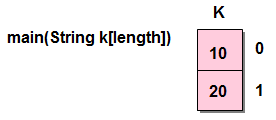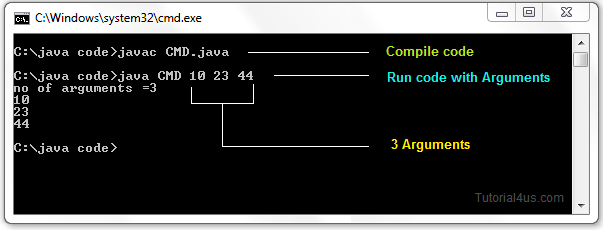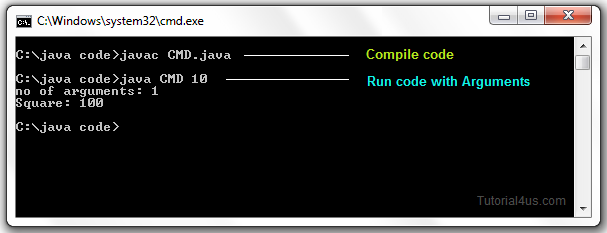# Command Line Arguments in Java

## Command Line Arguments in Java

If any input value is passed through the command prompt at the time of running of the program is known as command line argument by default every command line argument will be treated as string value and those are stored in a string array of main() method.

## Syntax for Compile and Run CMD programs

```Compile By -> Javac  Mainclass.java
Run By -> Java  Mainclass value1 value2  value3 ....................
```## Program Command Line Argument in Java

```class CommandLineExample
{
public static void main(String args[])
{
System.out.println("Argument is: "+args);
}
}
```

## Compile and Run above programs

```Compile By > Javac  CommandLineExample.java
Run By > Java  CommandLineExample Porter
```

## Output

```Argument is: Porter
```

## Example of command-line argument in java

```class SumDemo
{
public static void main(String args[])
{
System.out.println("Sum: "+args);
}
}
```

## Compile and Run above programs

```Compile By > Javac  SumDemo.java
Run By > Java  SumDemo 10 20
```

## Output

```Sum: 30
```

When the above statement is executing the following sequence of steps will take place.

• Class loader sub-system loads SumDemo along with Command line argument(10, 20) and in main memory.
• JVM takes the loaded class SumDemo along with Command line arguments (10, 20) and place the number of values in the length variable that is 2.
• JVM looks for main() that is JVM will place the Command in the main() in the form of string class that is.
•• Hence all the CMD line arguments of Java are sending to main() method available in the form of an array of object of String class (every CMD are available or stored in main method in the form of an array of object of String class).
• JVM calls the main() method with respect to load class SumDemo that is SumDemo.main().

## Accept command line arguments and display their values

```class  CMD
{
public static void main(String k[])
{
System.out.println("no. of arguments ="+k.length);
for(int i=0;i< k.length;i++)
{
System.out.println(k[i]);
}
}
}
```Note: Except + operator any numeric operation not allowed in command line arguments.

## Square of Number by reading value from command prompt.

```class squareDemo
{
int no, result;
void square(String s)
{
int no=Integer.parseInt(s);
result=no*no;
System.out.println("Square: " +result);
}
}
class CMD
{
public static void main(String args[])
{
System.out.println("no of arguments: "+args.length);
squareDemo  obj=new  squareDemo();
obj.square(args);
}
}
```